``

Mathematics is an equally important section for CTET, MPTET, KVS & DSSSB Exams and has even more abundant importance in some other exams conducted by central or state govt. Generally, there are questions asked related to basic concepts, Facts and Formulae of the Ratio and Proportion.

To let you make the most of Mathematics section, we are providing important facts related to the Ratio and Proportion. At least 1-2 questions are asked from this topic in most of the teaching exams. We wish you all the best of luck to come over the fear of the Mathematics section.

How to Overcome Exam Fever, Especially When You Fear Maths

## Ratio And Proportion

Ratio: Ratio is the comparison between two quantities in terms of their magnitudes. The ratio of two quantities a and b in some units, is the fraction a/b and we write it as a: b. In the ratio a:b, we call ‘a’ as the first term or antecedent and ‘b’, the second term or consequent.

Ex. The ratio 2:3 represents 2/3 with antecedent= 2, consequent = 3

Example: A type writer takes 8 hours to type 960 pages. How many pages will it type in 12 hours?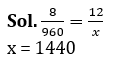Mathematics Study Notes For All Teaching Exams
Rule

• The multiplication or division of each term of a ratio by the same non-zero number does not affect the ratio.
• The comparison should always be done of the same quantity (of length, of weight etc.)

Ex. 2 : 3 = 4 : 6 = 8 : 9 = 10 : 15
Example: The two number are in ratio 2 : 3. If sum of the square two numbers is 20800, find the numbers?
Sol. 4x² + 9x²= 20800
13x² = 20800
x² = 1600
x = 40
Ist no. = 80, IInd no. = 120

Mathematics Quiz For CTET Exam : Attempt Daily Quizzes

Proportion: When two ratios are equal, then the four quantities involved in the two ratios are said to be proportional.

When a, b, c, d are in proportion, then a and d are called EXTREMES and b and c are called MEANS.

If a: b = c: d, we write, a: b :: c:d and say that a, b, c, d are in proportion. Here a and b are called extremes, and b and c are called means terms.

Thus a:b :: c:d ⇒ (a × d) = (b × c)
Ex. 2:3 :: 5:7 ⇒ (2 × 7)= (3 × 5)

Example: Which of the following numbers should be added to 13, 43, 23, and 73 So that they are in a proportion?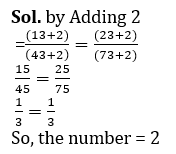How To Score 25+ Marks In Mathematics For CTET Exam?

Example: find the value of ‘a’ in the following proportion 36 : 108 : : x : 12.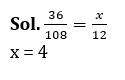Fourth proportional: If 2:3 :: 5:7, then 7 is called the fourth proportional to 2,3,5

Example: If a : b: c is 2 : 5 : 3 and c :  d : e is 2 : 3 : 5  then find a : b : c : d : e ?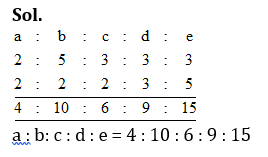Third proportional: If 2:3 :: 5:7, then 5 is called the third proportional to 2 and 3

Example: If A : B is 2 : 5, B: C is 3: 4, then find A : B : C.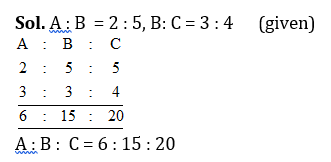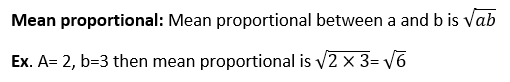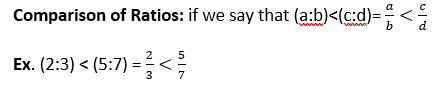Compounded ratio: The compound ratio of the ratios (a:b), (c:d), (e:f) is (ace : bdf)

Ex. (2:3), (5:7), (11:13)
= (2×5×11) : (3×7×13)
= 110 : 273
Duplicate ratio of (a:b) is (a²:b²)
Ex. Duplicate ratio of (2:3) is (2²:3²)
= (4:9)

Example: If P : Q  = 2 : 3 and Q : R  = 4 : 7 then (P + Q)² : (Q + R)² is

Sol.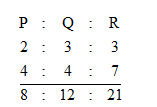(8 +12)²  : (12 +21)²
(20)² : (33)²
400: 1089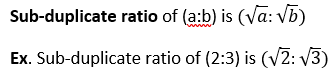Triplicate Ratio of (a:b) is (a³:b³)
Ex. Triplicate ratio of (2:3) is (2³:3³)
= (8:27)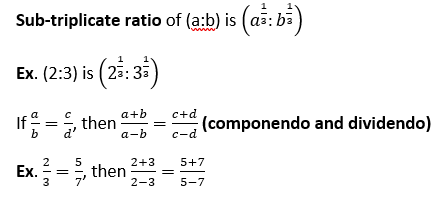Variation: Two quantities A and B may be such that as one quantity changes in value, the other quantity also changes in value bearing certain relationship to the change in the value of the first quantity.

Example: P : Q : R along completed a piece of work in 20, 40 and 30 days respectively. The ratio of the salary of each day is 5: 4: 3 respectively. If the total salary of Q is Rs.144, find total salary of P.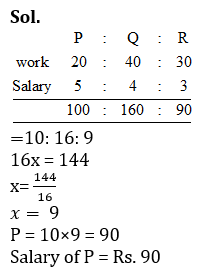•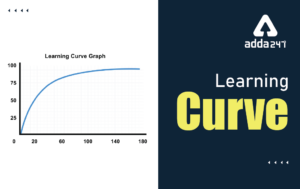Learning Curve - What is Learning Curve?...
•रस - परिभाषा, भे�...
•पठन कौशल- परिभा�...
•अलंकार - परिभाष�...
•Indian States and Capitals 2022: 28 Stat...
•New Education Policy(NEP) PDF - नई �...